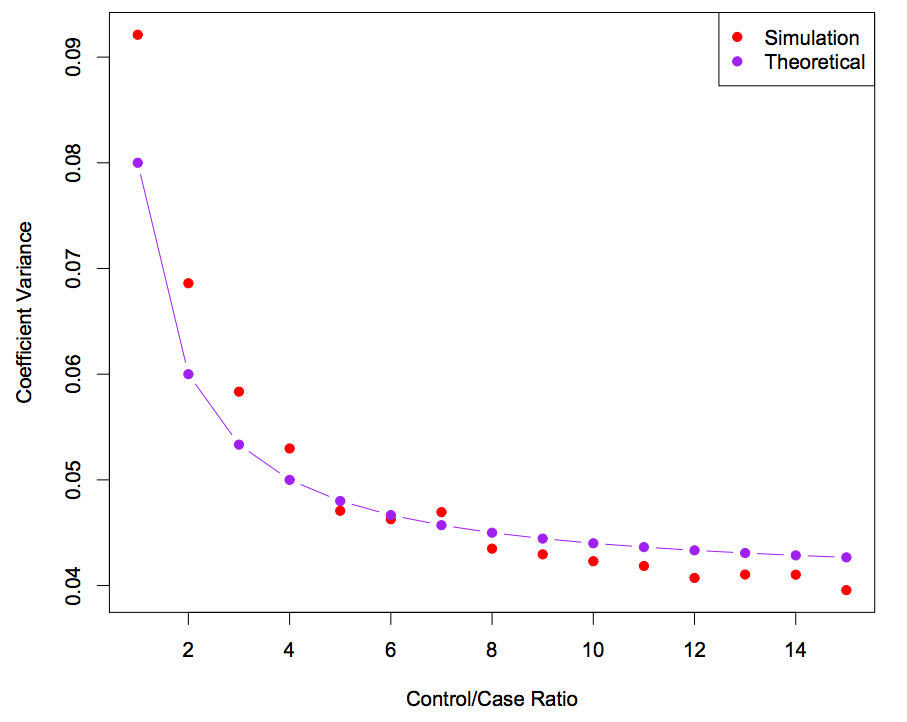# Case-control Sampling in Imbalanced Datasets

This problem was briefly introduced in STATS315A Applied Modern Statistics: Learning by the renowned Professor Trevor Hastie. Given that the imbalanced dataset problem is ubiquitous in data science, such as modeling click through rate or anomaly detection, I think the case-sampling trick was one of the most practical tricks I have learned in this class.

### Case-Control Sampling Use Case

The concept of case-control sampling originated in epidemiology, where a prospective study is often costly in time and money so a retrospective study is choose instead. Let’s take an example of studying a disease with 1% prevalence. The prospective study would be collecting data on a large group of people for a period of time. However, getting 1000 cases means following a group of 100,000 people, which is impractical. The retrospective study would be sampling cases and controls at different rates to form a dataset. Often cases are rare so they are all taken. Up to a few times of controls are taken as well.

### Intercept Transformation

However, the case-control sample has a much greater prevalence. Assuming we are modeling with logistic regression, we still have the correct regression coefficients $\beta$, but the intercept term $\beta_0$ is incorrect. We can correct the estimated intercept by the transformation (shameless stolen from lecture slide 4 of STATS315A) $$\hat{\beta}^\ast_0 = \hat{\beta}_0 + \text{log}\frac{\pi}{1-\pi} - \text{log}\frac{\pi^\ast}{1-\pi^\ast}$$ A more detailed proof can be found with Separate Sample Logistic Discrimination(Anderson et al. 1972)

### Diminishing return on coefficients variance

Sampling more negatives/controls does give us more data, which reduces the variance of the coefficients. However, since the number of positives/cases is fixed in the dataset, beyond some point, the variance in the coefficients mainly comes from the positives/cases. Sampling only more negatives/controls has a diminishing return effect, as shown by the following plot (shameless stolen from lecture slide 4 of STATS315A).### Extension to Modern Data Science Application

The idea of case-control sampling can be easily extend to modern data science application. When modeling click through rate, very often there is 1 positives (click-through) in more than 10000 data points (impression), which gives a control/case ratio of greater than 10000. With the same diminishing return effect as described in last paragraph, the large number of negatives doesn’t offer much other than wasting computing powers. By appling the case-control sampling method, We can undersample the negatives by taking 10-15 negatives per positives and achieve a similar performance as using the full dataset, but much faster computation.

See Local Case-Control Sampling: Efficient Subsampling in Imbalanced Data Sets(Fithian et al. 2014) for more advanced subsampling techniques that is consistent even when the model is misspecified.